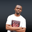Related Tags

d
communitycreator

# Approximation in D using math module methodsNDUKWE CHIDERA K.

### Overview

The D language has many built-in modules and libraries which must be imported into any program before such a program can access it. These modules usually provide the program into which a handful of methods can be imported.

The math module is one of such very important and vital modules that provides various methods used in mathematical evaluations.

A very common mathematical manipulation is approximation. The approximation can occur in different ways. Either we approximate a fractional value to the nearest value on the lower or higher side of such value.

### How to use the math method for approximations

To use the math method in a program, we should first import it at the top end of the program code before the main method:

import  std.math


With the statement above, our math module is ready to be used. Now start the approximations for which the D math module has three powerful methods that can be used. These methods are highlighted below:

• ceil(): The ceil method takes the float value passed to it as an argument and rounds it up to the next higher decimal value. For example, the ceil() method will approximate the value 4.4 up to 5.
• floor(): The floor() method, as the name implies, approximates or rounds off a fractional number that is a float value to the nearest lower number. For example, 4.4 will be rounded to 4.
• round(): The round() method, unlike the other two methods, have a decision to make. It looks at the value after the decimal point in the float number and checks if it is equal to or greater than 5, in this case, it rounds the value to the next higher one. Otherwise, it rounds it to the next lower one.

### Syntax

ceil(float_to_be_rounded);
float(float_to_be_rounded);
round(float_to_be_rounded);


### Parameter

Generally, these methods are similar in structure. They only accept one parameter, which is the float_to_be_rounded.

### Code

Let’s look at the code below:

import std.stdio;
import std.math;

void main(){
writeln("ceil value of 4.5 is: ",ceil(4.5));
writeln("floor value of 4.5 is: ",floor(4.5));
writeln("round value of 4.44 is: ",round(4.44));
writeln("round value of 4.45 is: ",round(4.5));
}

Demo

### Explanation

In the code snippet above,

• Line 1: We import the stdio module to enable us to display the output.
• Line 2: We import the math module from the standard library to allow us to use theceil(), round(), and floor() methods.
• Lines 4 to 9: We have the main function block.
• Lines 5 to 6: We can see the difference between floor and ceil as shown in the output because of their different effect on the same value 4.5 as indicated in the code.
• Lines 7 to 8: We pass the round() method to the arguments 4.44 and 4.5 to show the decision-making action of the method as is evident in the result.

RELATED TAGS

d
communitycreator

CONTRIBUTORNDUKWE CHIDERA K.
RELATED COURSES

View all Courses

Keep Exploring

Learn in-demand tech skills in half the time